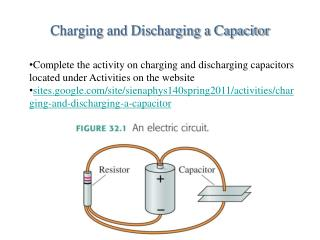# Charging and Discharging a Capacitor - PowerPoint PPT PresentationDownload PresentationCharging and Discharging a Capacitor

Download Presentation## Charging and Discharging a Capacitor

- - - - - - - - - - - - - - - - - - - - - - - - - - - E N D - - - - - - - - - - - - - - - - - - - - - - - - - - -
##### Presentation Transcript

1. Charging and Discharging a Capacitor • Complete the activity on charging and discharging capacitors located under Activities on the website • sites.google.com/site/sienaphys140spring2011/activities/charging-and-discharging-a-capacitor

2. Tactics: Using Kirchhoff’s loop law

3. EXAMPLE 32.1 A single-resistor circuit

4. EXAMPLE 32.1 A single-resistor circuit

5. Energy and Power The power supplied by a battery is The units of power are J/s, or W. The power dissipated by a resistor is Or, in terms of the potential drop across the resistor

6. EXAMPLE 32.4 The power of light

7. EXAMPLE 32.4 The power of light

8. Series Resistors • Resistors that are aligned end to end, with no junctions    between them, are called series resistors or, sometimes,    resistors “in series.” • The current I is the same through all resistors placed in    series. • If we have N resistors in series, their equivalent    resistance is The behavior of the circuit will be unchanged if the N series resistors are replaced by the single resistor Req.

9. EXAMPLE 32.7 Lighting up a flashlight

10. EXAMPLE 32.7 Lighting up a flashlight

11. Parallel Resistors • Resistors connected at both ends are called parallel    resistors or, sometimes, resistors “in parallel.” • The left ends of all the resistors connected in parallel are    held at the same potential V1, and the right ends are all    held at the same potential V2. • The potential differences ΔV are the same across all    resistors placed in parallel. • If we have N resistors in parallel, their equivalent    resistance is The behavior of the circuit will be unchanged if the N parallel resistors are replaced by the single resistor Req.

12. Series and Parallel Resistors

13. EXAMPLE 32.10 A combination of resistors

14. RC Circuits • Consider a charged capacitor, an open switch, and a    resistor all hooked in series. This is an RC Circuit. • The capacitor has charge Q0 and potential difference ΔVC  = Q0/C. • There is no current, so the potential difference across the    resistor is zero. • At t = 0 the switch closes and the capacitor begins to    discharge through the resistor. • The capacitor charge as a function of time is    where the time constant τ is

15. Applications

16. EXAMPLE 32.14 Exponential decay in an RC circuit

17. Junction Rule Circuits

18. Resistance, Voltage • Determine (a) the equivalent resistance of the circuit and (b) the voltage across each resistor. Circuits

19. Rank in order of brightness • Rank bulbs 1 through 6 in order of descending brightness. • Brightness is proportional to power • Now assume the filament in B6 breaks. Again rank the bulbs in order of descending brightness. Circuits

20. Practice Problems • Determine the equivalent resistance and the current through R1 for the circuits shown. Assume R1 = 10, R2 = 20 , and R1 = 30 , and the battery is 12 V. Circuits

21. Activities • Exploration of Physics • E&M • Resistive circuits • Do each of the 5 circuits – set all to 50 ohms • Calculate I, V, and P for each resistor and then check answers in the program Current & Resistance